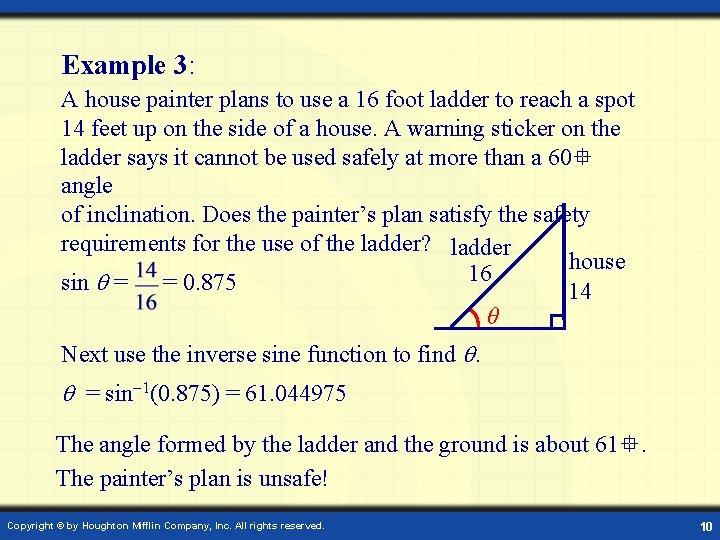Digital Lesson Trigonometric Applications and Models Trigonometric Functions

• Slides: 10Digital Lesson Trigonometric Applications and ModelsTrigonometric Functions on a Calculator Example 1: Calculate sin 40. Set the calculator in degree mode. Calculator keystrokes: sin 40 = Display: 0. 6427876 Example 2: Calculate cos 48. Calculator keystrokes: cos 48 = Display: 0. 669130606 Copyright © by Houghton Mifflin Company, Inc. All rights reserved. 2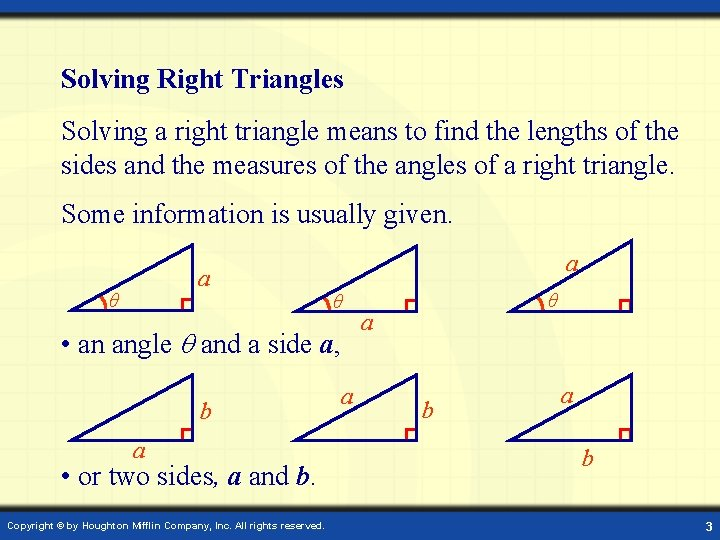Solving Right Triangles Solving a right triangle means to find the lengths of the sides and the measures of the angles of a right triangle. Some information is usually given. a θ • an angle and a side a, b a • or two sides, a and b. Copyright © by Houghton Mifflin Company, Inc. All rights reserved. a θ a b 3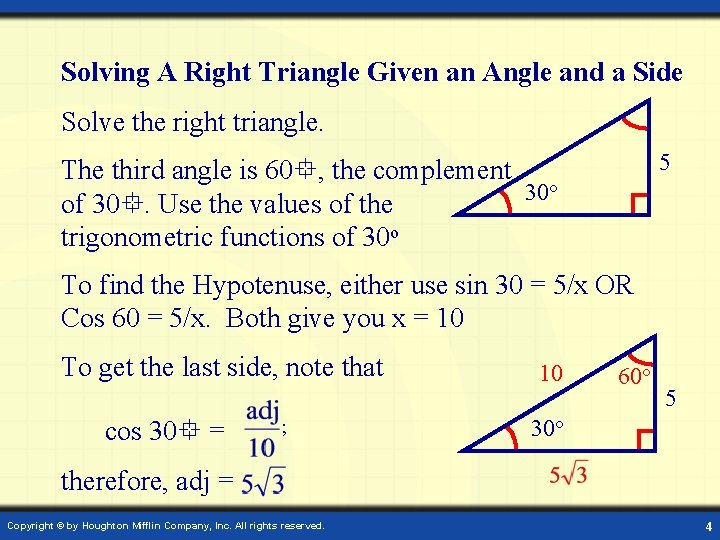Solving A Right Triangle Given an Angle and a Side Solve the right triangle. 5 The third angle is 60 , the complement ○ 30 of 30. Use the values of the trigonometric functions of 30 o To find the Hypotenuse, either use sin 30 = 5/x OR Cos 60 = 5/x. Both give you x = 10 To get the last side, note that cos 30 = ; 10 60○ 5 30○ therefore, adj = Copyright © by Houghton Mifflin Company, Inc. All rights reserved. 4Example 1: A bridge is to be constructed across a small river from post A to post B. A surveyor walks 100 feet due south of post A. She sights on both posts from this location and finds that the angle between the posts is 73. Find the distance across the river from post A to post B. x Post B Post A Use a calculator to find tan 73 o = 3. 27. 100 ft. ○ opp 73 3. 27 = tan 73 = = adj It follows that x = 327. The distance across the river from post A to post B is 327 feet. Copyright © by Houghton Mifflin Company, Inc. All rights reserved. 5Inverse Trigonometric Functions on a Calculator Labels for sin 1, cos 1, and tan 1 are usually written above the sin, cos, and tan keys. Inverse functions are often accessed by using a key that maybe be labeled SHIFT, INV, or 2 nd. Check the manual for your calculator. Example: Find the acute angle for which cos = 0. 25. Calculator keystrokes: (SHIFT) cos 1 0. 25 = Display: 75. 22487 Copyright © by Houghton Mifflin Company, Inc. All rights reserved. 6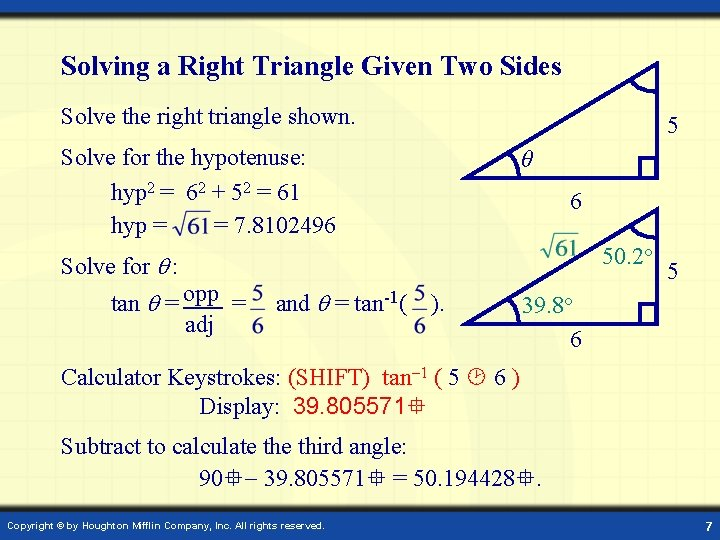Solving a Right Triangle Given Two Sides Solve the right triangle shown. 5 Solve for the hypotenuse: hyp 2 = 62 + 52 = 61 hyp = = 7. 8102496 θ 6 50. 2○ Solve for : tan = opp = adj and = tan-1( ). 5 39. 8○ 6 Calculator Keystrokes: (SHIFT) tan 1 ( 5 6 ) Display: 39. 805571 Subtract to calculate third angle: 90 39. 805571 = 50. 194428. Copyright © by Houghton Mifflin Company, Inc. All rights reserved. 7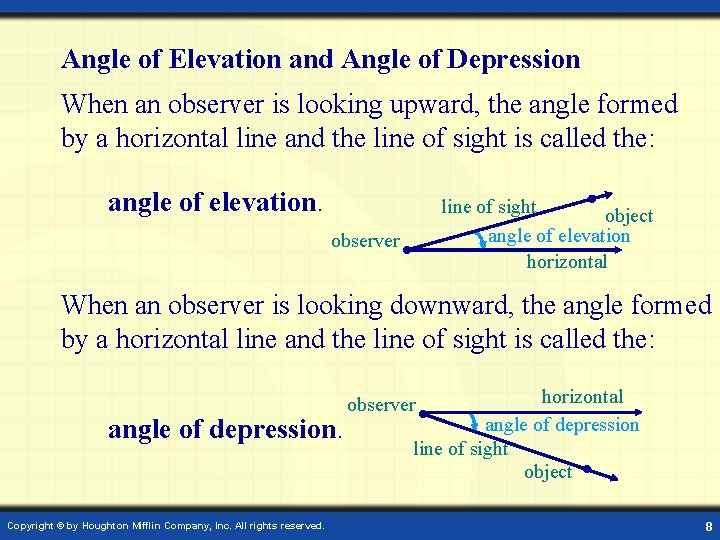Angle of Elevation and Angle of Depression When an observer is looking upward, the angle formed by a horizontal line and the line of sight is called the: angle of elevation. line of sight object angle of elevation horizontal observer When an observer is looking downward, the angle formed by a horizontal line and the line of sight is called the: angle of depression. Copyright © by Houghton Mifflin Company, Inc. All rights reserved. horizontal angle of depression line of sight object observer 8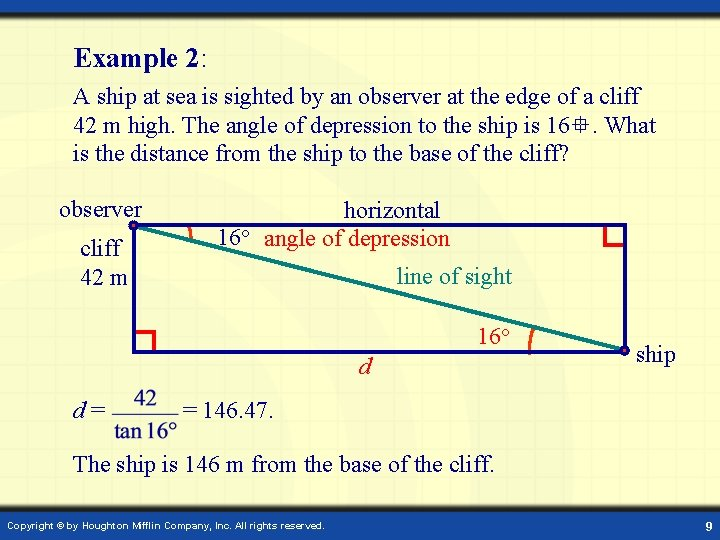Example 2: A ship at sea is sighted by an observer at the edge of a cliff 42 m high. The angle of depression to the ship is 16. What is the distance from the ship to the base of the cliff? observer cliff 42 m horizontal 16○ angle of depression line of sight 16○ d d= ship = 146. 47. The ship is 146 m from the base of the cliff. Copyright © by Houghton Mifflin Company, Inc. All rights reserved. 9